# 10 Position scales and axes

## 10.1 Exercises

1. The following code creates two plots of the mpg dataset. Modify the code so that the legend and axes match, without using faceting!

``````fwd <- subset(mpg, drv == "f")
rwd <- subset(mpg, drv == "r")

ggplot(fwd, aes(displ, hwy, colour = class)) +
geom_point() +
scale_color_discrete(limits = c("compact", "midsize", "minivan", "subcompact",
"2seater", "suv")) +
coord_cartesian(xlim = c(1, 8),
ylim = c(15, 50))``````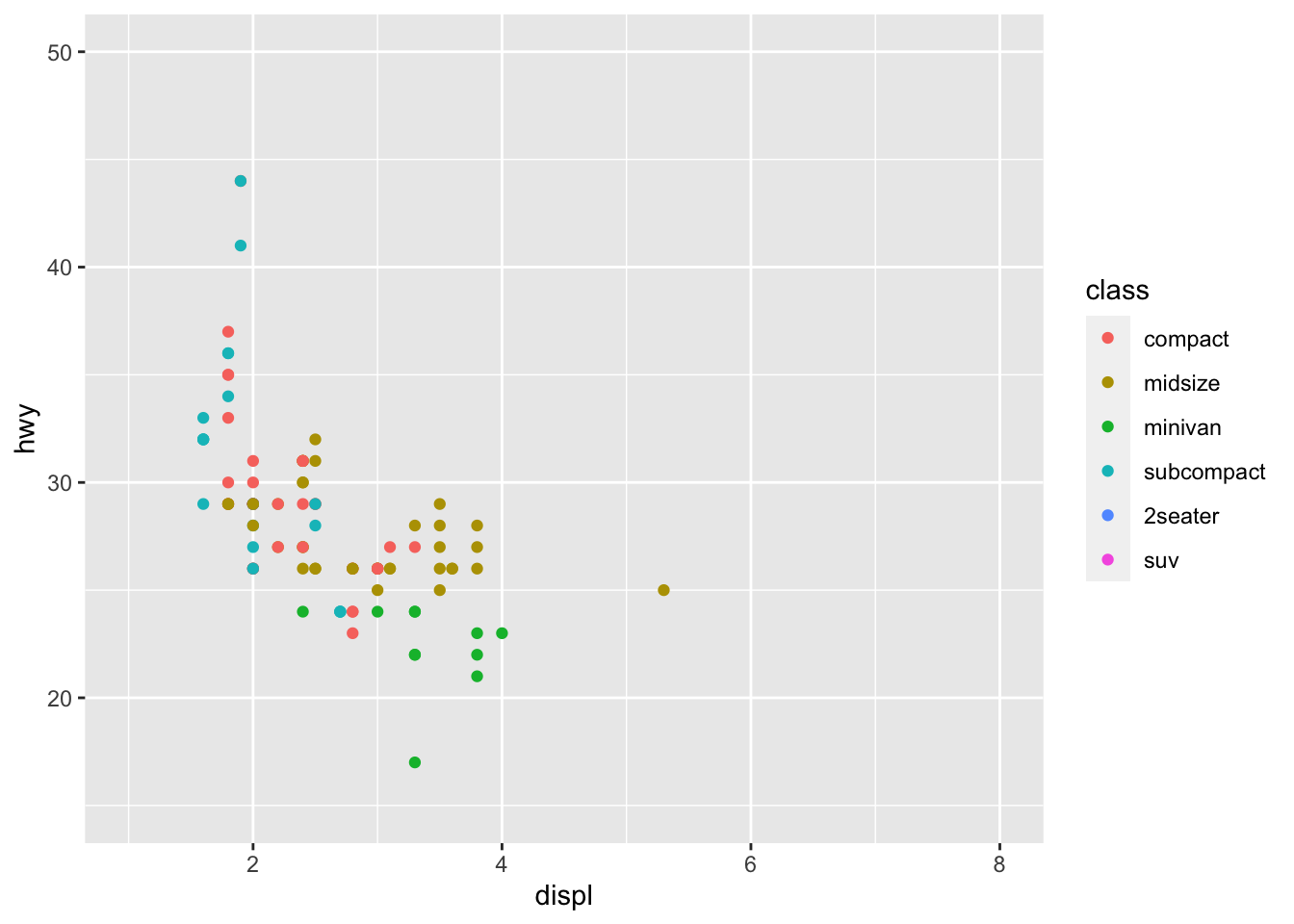``````
ggplot(rwd, aes(displ, hwy, colour = class)) +
geom_point() +
scale_color_discrete(limits = c("compact", "midsize", "minivan", "subcompact",
"2seater", "suv")) +
coord_cartesian(xlim = c(1, 8),
ylim = c(15, 50))``````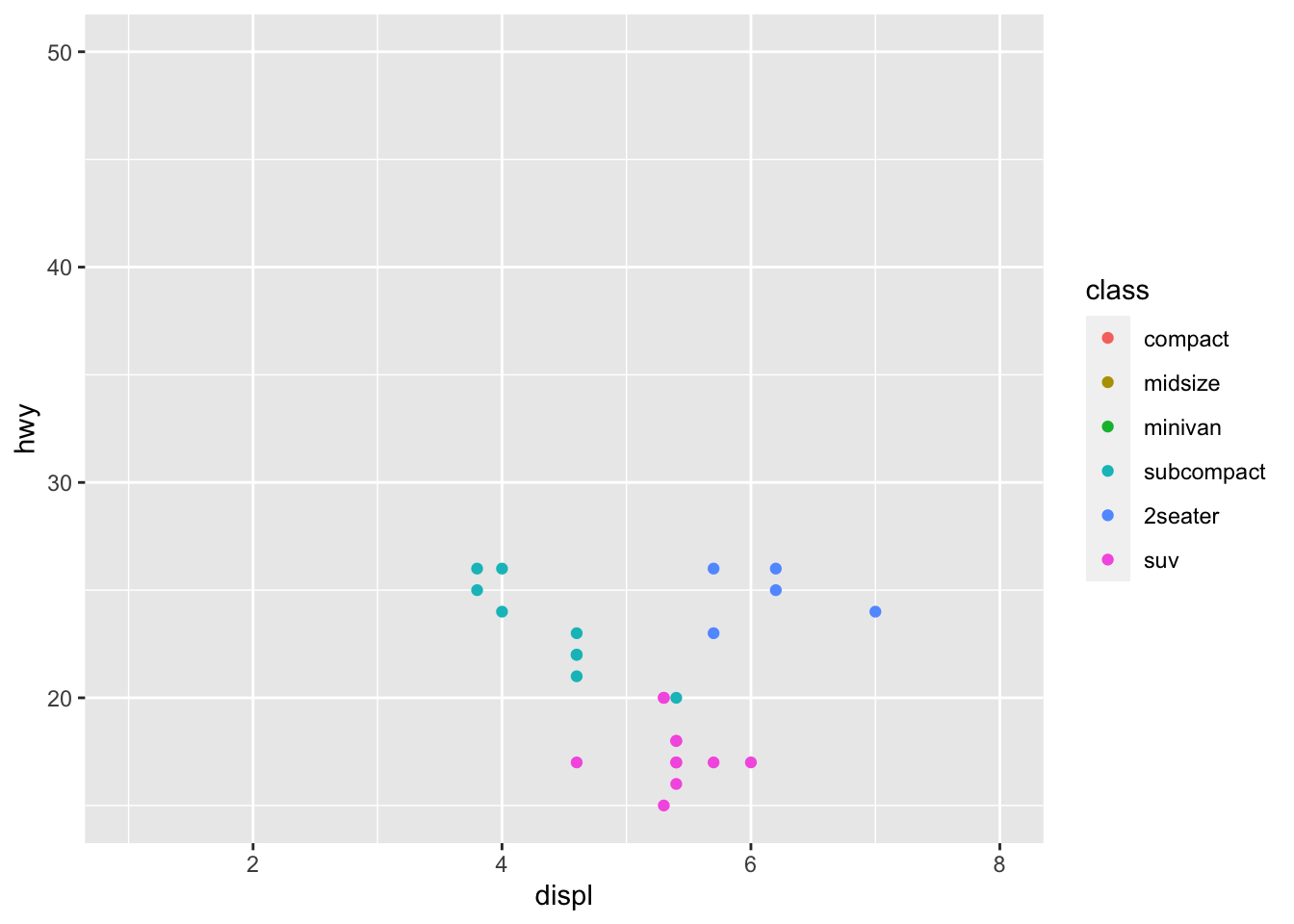• We can make the legend and axes match by manually setting the limits of color.

2. What happens if you add two `xlim()` calls to the same plot? Why?

``````ggplot(fwd, aes(displ, hwy, colour = class)) +
geom_point() +
xlim(2, 5) +
xlim(3, 4)
#> for 'x', which will replace the existing scale.
#> Warning: Removed 75 rows containing missing values
#> (geom_point).``````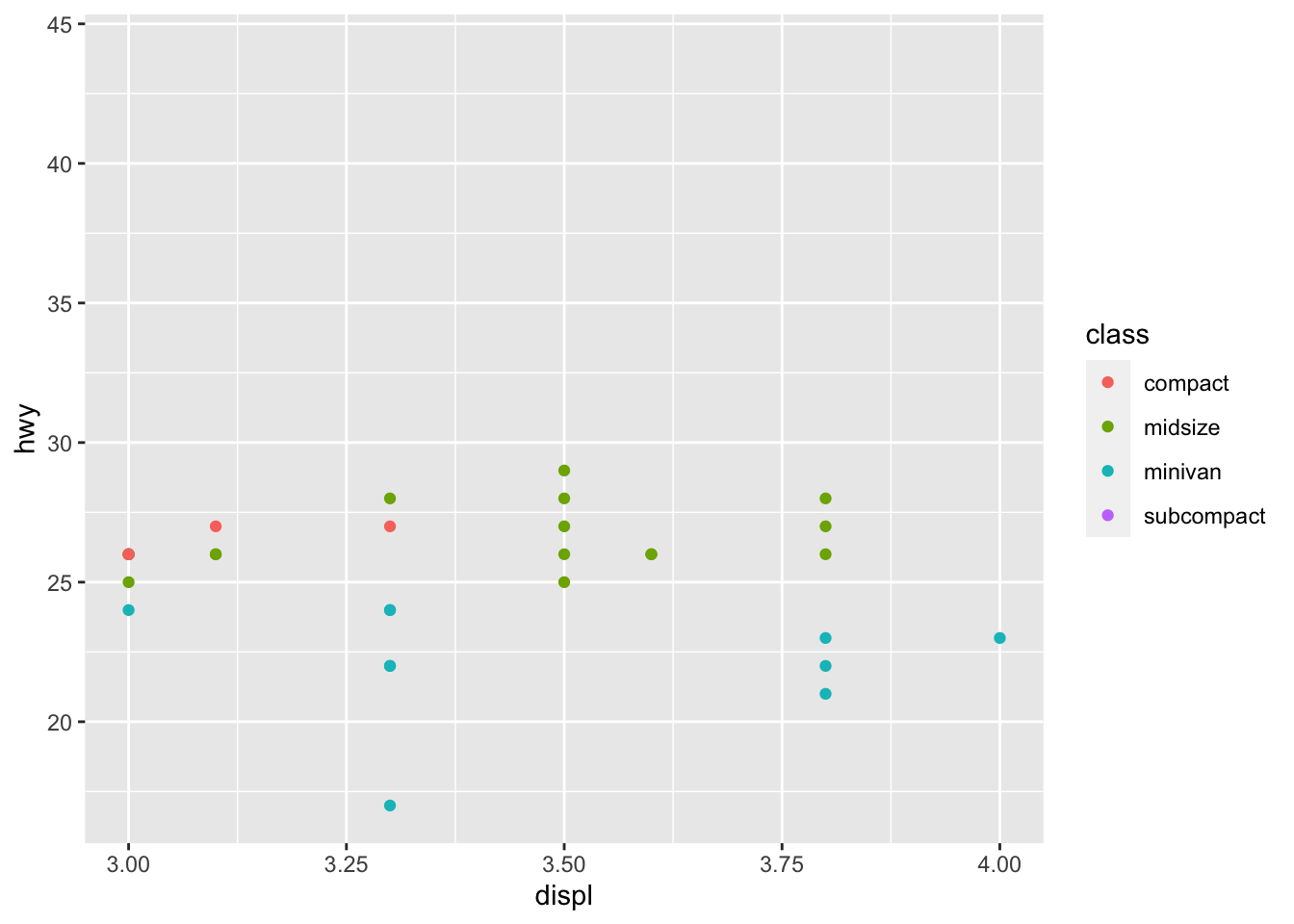• You get a very informative message: `Scale for 'x' is already present. Adding another scale for 'x', which will replace the existing scale.` I’m guessing the reason is ggplot evaluates the code top-down. Since the second `xlim` call happens after the first `xlim`, it replaces the first `xlim`.

3. What does `scale_x_continuous(limits = c(NA, NA))` do?

``````ggplot(fwd, aes(displ, hwy, colour = class)) +
geom_point() +
scale_x_continuous(limits = c(NA, NA))``````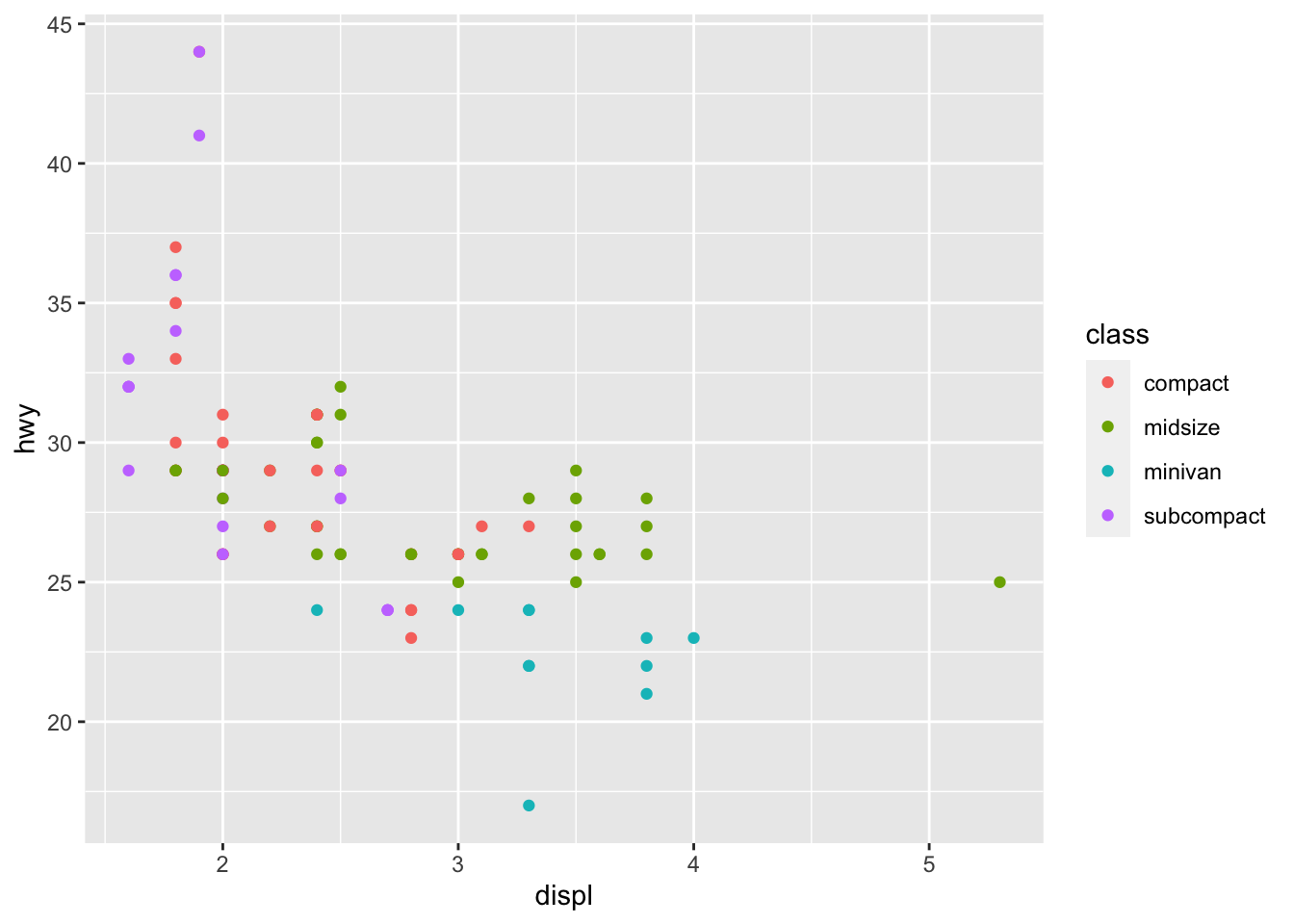• It doesn’t change the limits because, according to the help page, “use NA to refer to the existing minimum or maximum”. This means that we are setting the limits to be from the existing minimum to the existing maximum.

4. What does `expand_limits()` do and how does it work? Read the source code.

``?expand_limits``
• According to the help page, “it ensures limits include a single value, for all panels or all plots”. It is a wrapper around `geom_blank()` and makes it easy to add such values.

## 10.2 Exercises

1. Recreate the following graphic:

``````ggplot(mpg, aes(displ, hwy)) +
geom_point(size = 3) +
scale_x_continuous("Displacement", labels = scales::unit_format(suffix = "L")) +
scale_y_continuous(quote(paste("Highway ", (frac(miles, gallon))))) ``````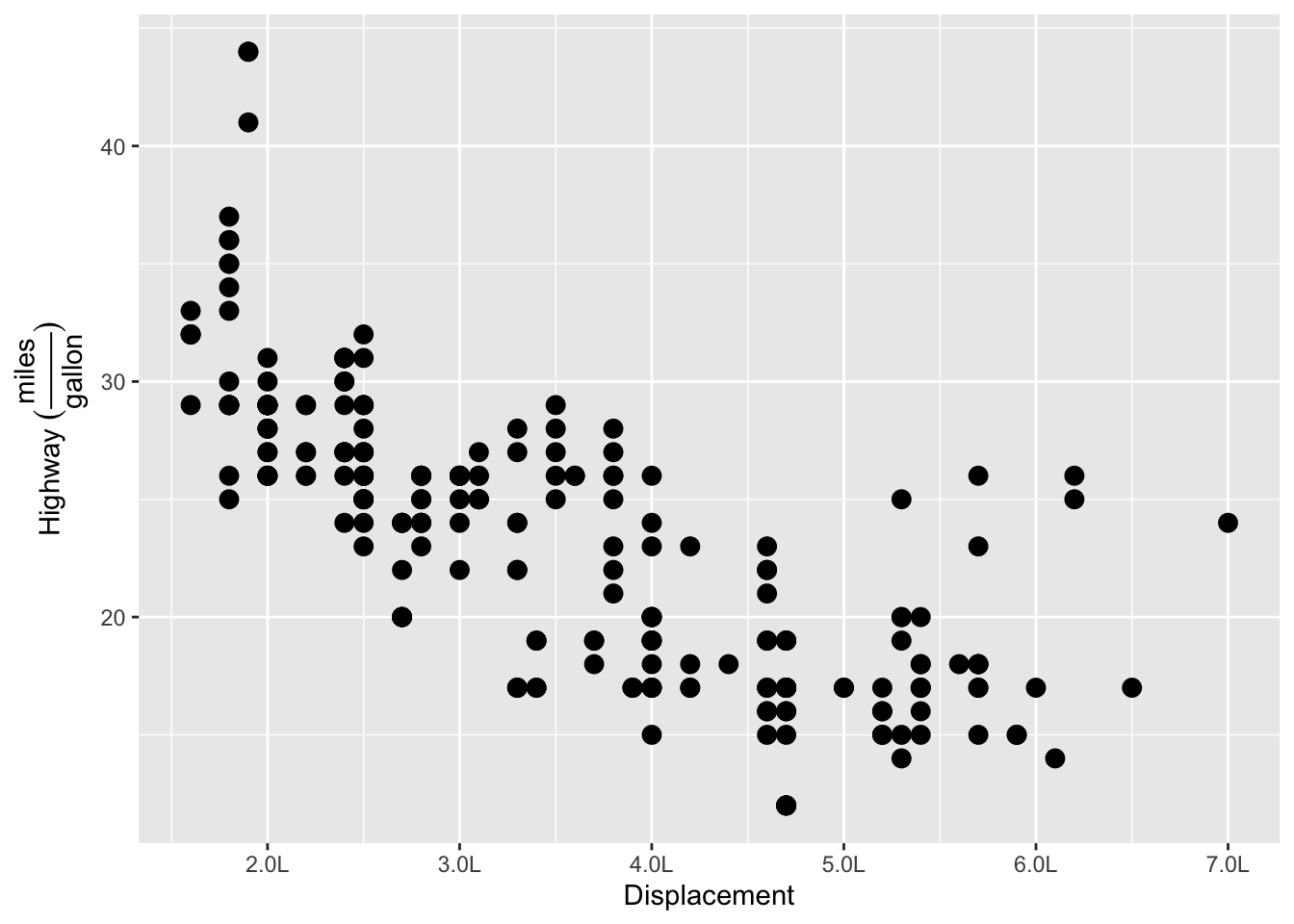2. List the three different types of object you can supply to the `breaks` argument. How do `breaks` and `labels` differ?

According to the help page, you can supply NULL, transformation object (from `waiver()`), a numeric vector, and a function that takes limits as input and returns breaks as output. - The `labels` argument takes in a character vector instead of a numeric vector, which `breaks` accepts. Also, it accepts a function that takes breaks as input (instead of limits) and returns labels as output (instead of breaks).

3. What label function allows you to create mathematical expressions? What label function converts 1 to 1st, 2 to 2nd, and so on?

• `label_math()` allows you to create mathematical expressions.
• `label_ordinal()` allows you to label ordinal numbers (1 to 1st, 2 to 2nd, 3 to 3rd and so on)